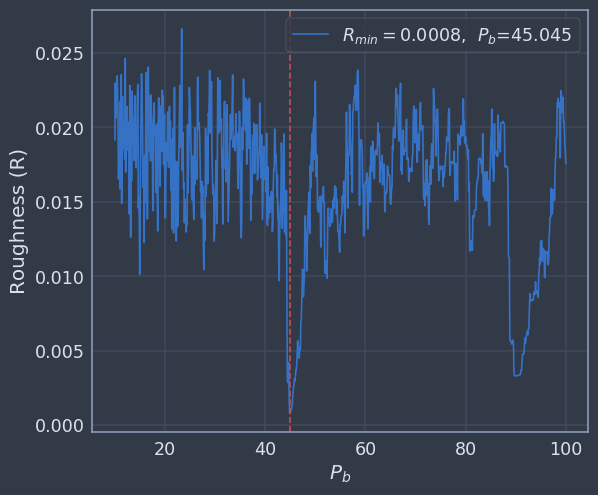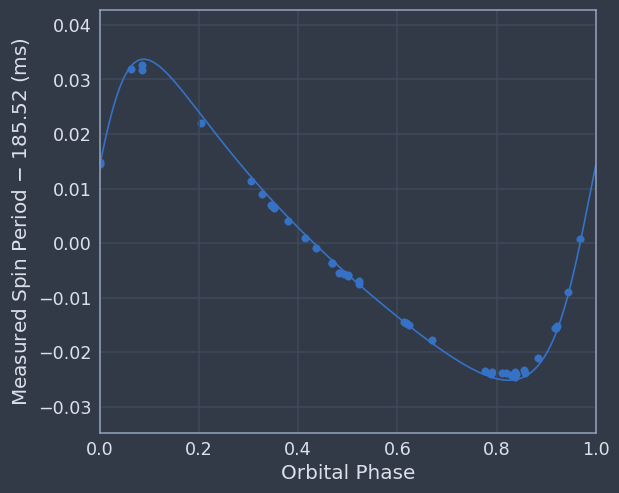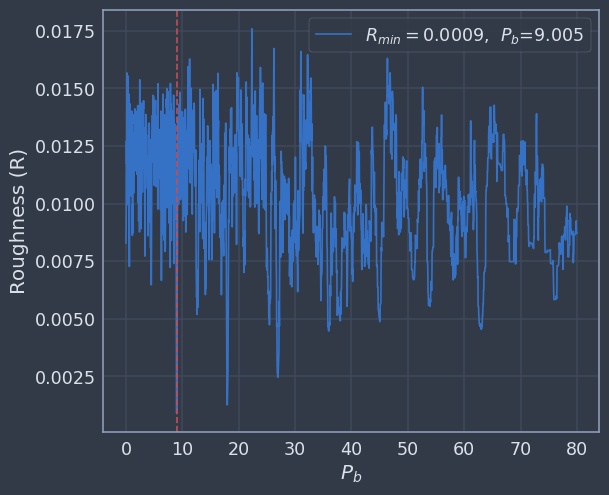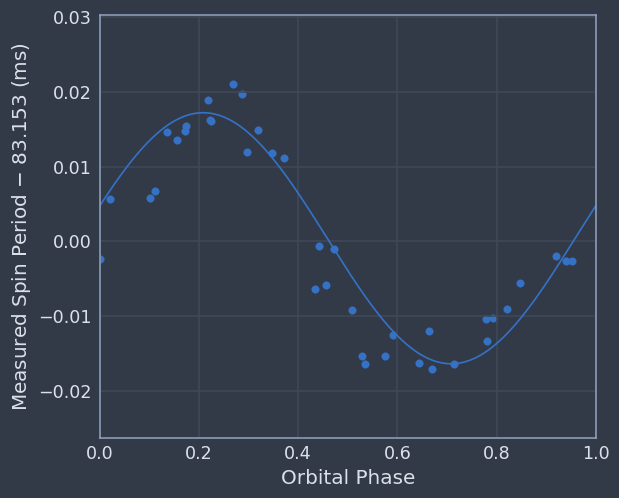In :
%matplotlib inline
import numpy as np
from orbit_functions import *
from jupyterthemes import jtplot
jtplot.style('chesterish')


# 1) Computing "Roughness" & Solving for Pb¶

In order to solve for the orbit of a pulsar, we must first use measured spin period values to get an initial value for orbital period (Pb) -- the amount of time it takes for the pulsar to orbit its companion once. To solve for orbital period, you will need to create a function following instructions from Section 2.1 Bhattacharyya & Nityananda (2008); http://adsabs.harvard.edu/abs/2008MNRAS.387..273B.

### 1.1) Skim Section 2.1 & Load Data¶

In :
epoch, period = np.loadtxt('1930.txt',dtype='float',unpack=True)


### 1.2) Compute Roughness¶

Write a function that computes roughness (R; see Equation 1). Following along with the hints below might help!

In :
# Make an array of values that represents 'time' since the first epoch.
time = epoch - epoch

In :
# Using a trial orbital period (Pb = 10 days) make another array of orbital phases. You may
# want to use the '%' (modulo) function since the range of orbital phase is from 0-1.
Pb = 10
phases = time%Pb / Pb

In :
# Find indices that sort the orbital phase array (look up np.argsort). Use these indices to
# sort period measurements by orbital phase.
sort = np.argsort(phases)

sorted_phases = phases[sort]
sorted_periods = period[sort]

In :
# Calculate roughness using Equation 1.
def roughness(epochs, periods, Pb):

R = 0

Pb = Pb
time = epoch - epoch
phases = (time/Pb)%1.0

sort = np.argsort(phases)

sorted_phases = phases[sort]
sorted_periods = period[sort]

for i in range(len(sorted_periods) -1):

R += (sorted_periods[i] - sorted_periods[i+1])**2

return R


In :
# Now generalize your code above! Write a function that computes R given three inputs:
# epochs (array), periods (array), trail Pb (float value)
R = roughness(epoch, period, Pb = 10)

print R

0.019160140514


### 1.3) Solve for Pb¶

Using your function, compute R for trial Pb values between 10-100 days. What Pb value minimizes roughness? To increment trial Pb, you can either follow the method outlined in Bhattacharyya & Nityananda (2008) or iterate over progressively smaller linear ranges to zero in on a minimum R value.

In :
# Decide how to increment Pb values and compute R for each one, saving result in a list/array.
R_list = []
a = np.linspace(10,100,1000)
for i in a:
R_list.append(roughness(epoch, period, Pb = i))

print a[np.where(np.array(R_list) == np.min(R_list))]
print np.min(R_list)

plt.plot(a, R_list, label = r'$R_{min}=$%.4f,  $P_b$=%.3f'%(np.min(R_list),a[np.where(np.array(R_list) == np.min(R_list))]))
plt.xlabel(r'$P_b$')
plt.ylabel('Roughness (R)')
plt.axvline(x = a[np.where(R_list == np.min(R_list))], color = 'r', linestyle = '--')
plt.legend()

[ 45.04504505]
0.000798415479208

Out:
<matplotlib.legend.Legend at 0x7f7d4e2979d0>In :
# Plot trial Pb vs. Roughness or find another way to determine the best Pb value.


# 2) Solving the Orbit¶

Make educated guesses to improve t0, a1, om, ecc and p0 parameters. We will use your current best parameters to convert MJD to orbital phase and plot measured spin periods versus orbital phase. The blue line represents your model prediction and individual points represent measured values. The 'per_vs_phi' function will produce a plot so that you can see your progress and improve your binary solution. Solve the orbit!

In :
# Read data file

pb = 45.045
t0 = np.min(epochs)
a1 = 90.0
om = -70
ecc = 0.4
p0 = 185.520268491

input_params = [p0,pb,a1,t0,om,ecc]

# Convert epochs to orbital phase (mean anomaly) and sort
phases = ((epochs-t0)/pb)%1.0
inds = np.argsort(phases)
sorted_periods = periods[inds]
sorted_phases = phases[inds]
#plt.scatter(sorted_phases, sorted_periods)

per_vs_phi(input_params,sorted_phases,sorted_periods)

MASS FUNCTION:  0.384569229513# 3) A New Discovery, J1017-15 (if time)¶

Repeat the full process above to get initial binary parameters for another pulsar, J1017-15, recently discovered in the GBNCC survey. You can find the discovery plot at http://astro.phys.wvu.edu/GBNCC/.

In :
epoch, period = np.loadtxt('1017.txt',dtype='float',unpack=True)

In :
R_list = []
a = np.linspace(0,80,2000)
for i in a:
R_list.append(roughness(epoch, period, Pb = i))

print a[np.where(np.array(R_list) == np.min(R_list))]
print np.min(R_list)

plt.plot(a, R_list, label = r'$R_{min}=$%.4f,  $P_b$=%.3f'%(np.min(R_list),a[np.where(np.array(R_list) == np.min(R_list))]))
plt.xlabel(r'$P_b$')
plt.ylabel('Roughness (R)')
plt.axvline(x = a[np.where(R_list == np.min(R_list))], color = 'r', linestyle = '--')
plt.legend()

[ 9.00450225]
0.000892618777997

/usr/local/lib/python2.7/dist-packages/ipykernel/__main__.py:8: RuntimeWarning: divide by zero encountered in divide
/usr/local/lib/python2.7/dist-packages/ipykernel/__main__.py:8: RuntimeWarning: invalid value encountered in divide
/usr/local/lib/python2.7/dist-packages/ipykernel/__main__.py:8: RuntimeWarning: invalid value encountered in remainder

Out:
<matplotlib.legend.Legend at 0x7f7d49e0e990>In :
pb = 9.005
t0 = np.min(epoch)
a1 = 25
om = -75
ecc = 0.0
p0 = 83.1534080263

a = np.linspace(-100,100,20)

input_params = [p0,pb,a1,t0,om,ecc]

# Convert epochs to orbital phase (mean anomaly) and sort
phases = ((epoch-t0)/pb)%1.0
inds = np.argsort(phases)
sorted_periods = period[inds]
sorted_phases = phases[inds]
#plt.scatter(sorted_phases, sorted_periods)

per_vs_phi(input_params,sorted_phases,sorted_periods)

MASS FUNCTION:  0.206249439999In [ ]:


In [ ]:


In [ ]: